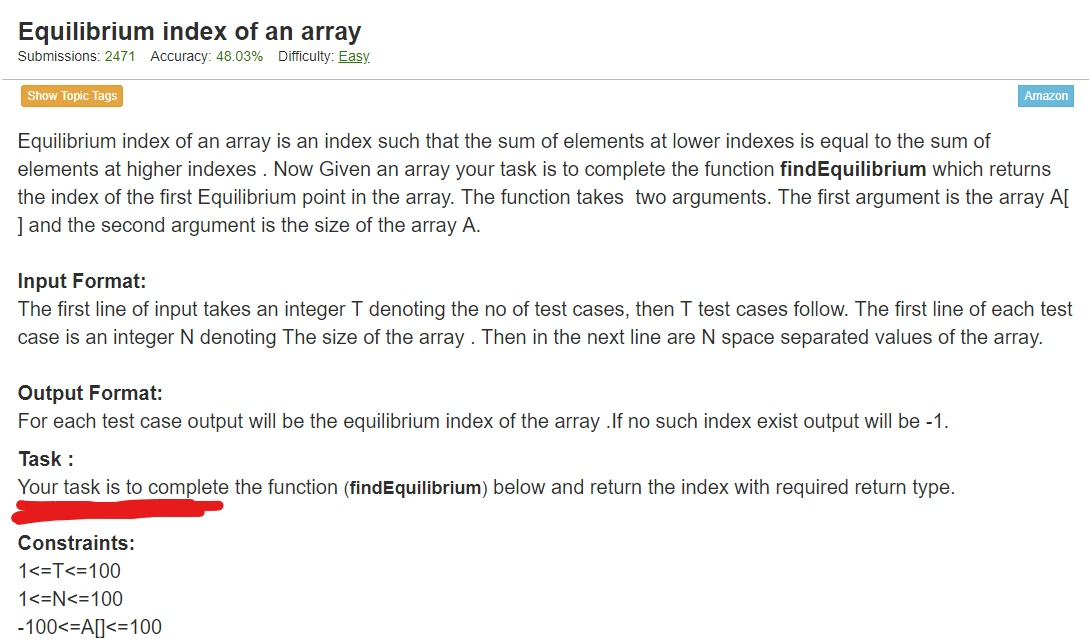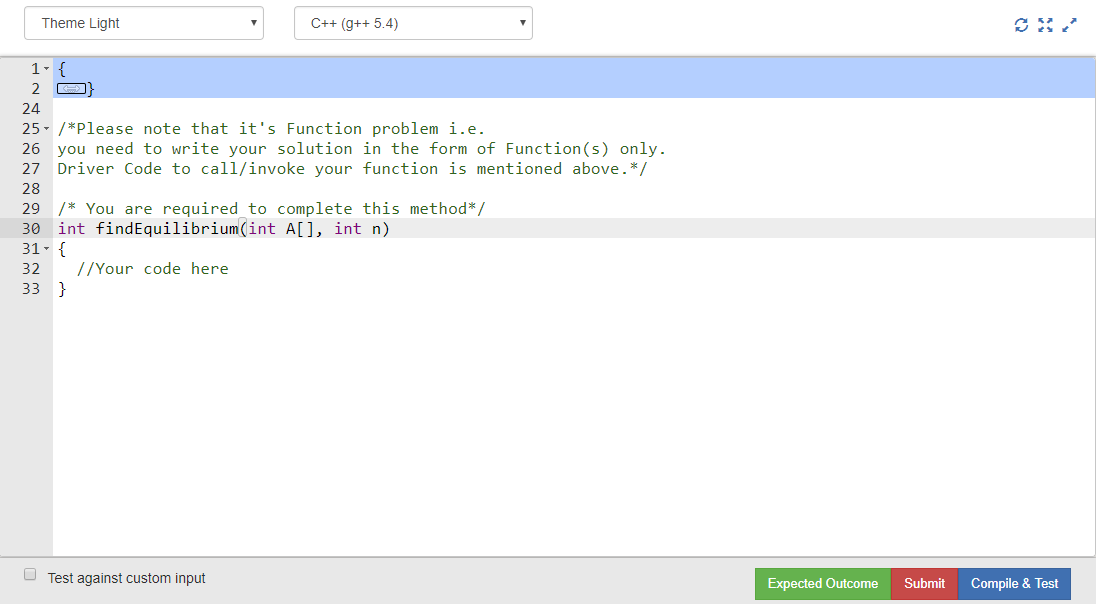# How to attempt Function Coding Questions?

Function Coding Questions are similar to normal coding questions apart from the fact that one does not need to read input and print output from standard I/O while writing solutions.

In function coding question, one just needs to complete a function already written in the code editor. You need to work on inputs provided as a parameter to this function and instead of printing the result you will have to return it from the function.

Let’s take the example of a sample function problem: Equilibrium Index of an Array. The pattern of question is almost the same as that of normal programming questions. In function problems, you can notice an additional information provided as “Task” after the output format which clearly explains that the user only needs to complete a function and return the result from it.You can also see that in the code editor for this question there is already a function written with name findEquilibrium().This function accepts an array A[] and an integer n which denotes the size of the array A[]. You can see in the above image that the body of this function is empty. You need to write your complete code inside the body of this function and return the result from it without writing a separate main() function. You must assume that there is already a main() function written which is invoking this function and write your program accordingly.

Below is the complete working solution for the above question:

 `/*Please note that it's Function problem i.e. ` `you need to write your solution in the form of Function(s) only. ` `Driver Code to call/invoke your function is mentioned above.*/` ` `  `/* You are required to complete this method*/` `int` `findEquilibrium(``int` `A[], ``int` `n) ` `{ ` `    ``// Your code here ` `    ``int` `i, y, f, j, x; ` `    ``for` `(i = 1; i < n; i++) { ` `        ``y = 0; ` `        ``x = 0; ` `        ``for` `(j = 0; j < i; j++) { ` `            ``y = y + A[j]; ` `        ``} ` `        ``for` `(j = i + 1; j < n; j++) { ` `            ``x = x + A[j]; ` `        ``} ` `        ``if` `(x == y) { ` `            ``f = 1; ` ` `  `            ``// returning answer ` `            ``return` `(i); ` `            ``break``; ` `        ``} ` `        ``else` `{ ` `            ``f = 0; ` `        ``} ` `    ``} ` `    ``if` `(f == 0) { ` `        ``// returning answer ` `        ``return` `(-1); ` `    ``} ` `} `

Important things to remember

• One should assume that a main() function is already present which is invoking the current function and should not write a separate main() function.
• Use inputs as provided in the function parameters.
• Return result from the function instead of printing it.

Attention reader! Don’t stop learning now. Get hold of all the important DSA concepts with the DSA Self Paced Course at a student-friendly price and become industry ready.

My Personal Notes arrow_drop_upCheck out this Author's contributed articles.

If you like GeeksforGeeks and would like to contribute, you can also write an article using contribute.geeksforgeeks.org or mail your article to contribute@geeksforgeeks.org. See your article appearing on the GeeksforGeeks main page and help other Geeks.

Please Improve this article if you find anything incorrect by clicking on the "Improve Article" button below.

Article Tags :

2

Please write to us at contribute@geeksforgeeks.org to report any issue with the above content.